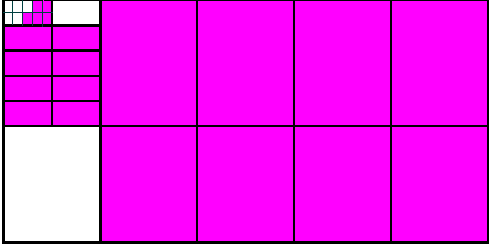Why?

Teaching decimals can sometimes be a difficult concept to teach and like many topics, students can often possess a number of misconceptions in this area. This lesson has been designed to address many of these misconceptions such as whole number thinking, longer is larger and shorter is larger. It introduces students to decimats and is designed for use with middle and upper primary students.

What?

The lesson introduces students to the decimat where they begin to make sense of decimal size and decimal place value as well as use fractional language to describe decimals. A decimat is a proportional model that represents the size of decimals as part of a whole. It provides students a visual representation and the way the lesson is designed, allows students to consolidate their values as a decimal, fraction and representation.The decimat consists of a large rectangle representing one whole. This is then broken up into ten parts representing tenths. Each tenth is also broken up into ten parts representing hundredths and each hundredth into thousandths.

The students then shade various parts of the decimat to show their decimal values.When using decimats, students are always encouraged to write the value as a decimal as well as a fraction in order to consolidate the understanding of decimal place value.

How?

Before introducing students to the decimats game, assign them the decimals comparison test in order to determine what, if any, misconceptions students have about decimals. It is important to iron out the common misconception of longer is larger and both the test and the task will work together to help students overcome this.

You may introduce the decimats to them slowly by only providing them with one representing tenths, or tenths and hundredths and once they have shown competence with these, you can then introduce the full decimat. When students are doing the task, it is important for them to complete each turn with a different colour in order to ensure that they can keep track of each turn. This will also make it easier for both the students and the teacher to check the work for any errors. Ensuring that the values are written both as a decimal and as a fraction is also extremely important because it establishes the link between the decimal and its equivalent fractional value.

After confidence has been established, encourage students to begin further dividing their tenths into hundredths and thousandths to continue to build understanding.

The Decimats game is designed to be used for an entire lesson and not just as a warm up. The more it is played over a decimals unit, the greater the likelihood that they will more easily make sense of decimals. Once the students are familiar with this model, they can then use it to compare the size of decimals by shading blank decimats and comparing the area.

Dig Deeper

For more information, the attached lesson plan shows the relevant links to the Australian Curriculum and highlights the related content and outcomes as listed in the Australian Mathematics F–10A syllabus.

Recent Posts